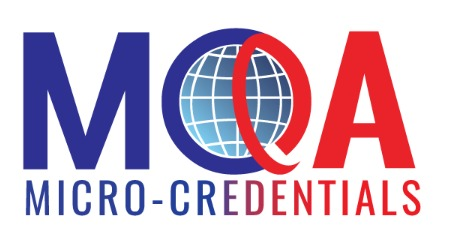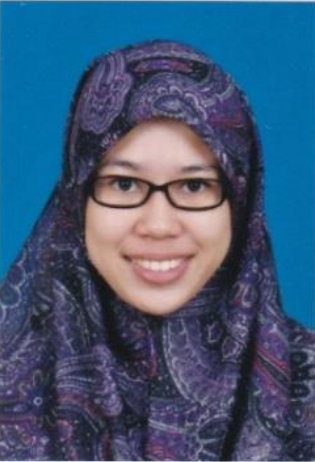# Discrete Mathematics4 Weeks Information and Communication Te Course Credit : 3
Micro-Credentials Language of Delivery : English
Share on
• 1 - Set
This topic explains the definition of set in discrete mathematics. The concept of a ‘set’ is one of the basic concepts of mathematics. It discusses the concepts of set, set equality, Venn diagram, subset, power set, set operations, generalized union and intersection, partition, and Cartesian product.

Topic Outcomes

1. To define the main notions and definitions.
2. To discuss the usage of notions in linguistics
• 2 - Relation
Relationship between elements of sets occur in many concepts. Relationships between elements of sets represented using the structure called a relation, which is just a subset of the Cartesian product of the sets. This topic looks at the general concept of a relation and the various ways of visualizing relations.

Topic Outcomes

1. To define the concept of relation, inverse relation, composition of relations, relation on a set, and digraph.
2. To recognize the partial order and equivalence relation.
• 3 - Function
This topic explains the definition of function in discrete mathematic. Function is a specific relation that is important to be used in computer science programming. It discusses the concept of function, graph function, types of function, inverse function, function composition, and binary and unary operators.

Topic Outcomes

1. To describe the concept and the use of function.
2. To identify the types of function and how function works in different perspective.
• 4 - Sequence and Strings
This topic explains the definition of sequence, strings, and the operators that involve in the programming of computer science. A sequence can be represented in ordered list of element (integers or characters) and usually the values are infinite. This topic discusses the details about the concept of sequence and strings.

Topic Outcomes

1. To explain the definition of sequence and strings.
2. To identify the operations that involve sequence and strings.
• 5 - Propositional Logic
It introduces some basic concepts of logical methods in mathematics that can be used to prove the validity of mathematical statements. In computer science, logic is used to verify the correctness of computer programs. Propositional logic is a combination of logic symbols to generate a conclusion from experiments and observations done by people. It represents any statement based on logic and symbols.

Topic Outcomes

1. To define the concept and meaning of propositions.
2. To identify a few types of propositions and apply operations on it.
3. To apply the propositions to the problem of computer programs.
• 6 - Predicate Logic
This topic relates the propositional logic to another type of logic called the predicate logic. Predicate logic is one of such logic that is able to express the issue in a more understandable way. Predicate logic is arguably the most important knowledge in representation language. It is a well-defined syntax, semantics, and rules of inference.

Topic Outcomes

1. To define the concept of the predicate logic.
2. To use the predicate logic concept in the logical statements.
3. To generate formal logic to represent problems in computer science.
• 7 - Integer
This topic discusses the basic form of discrete mathematics called integer. Integer is all about numbers and is related to operations to measure something. The discussion in this topic is based on number theory and the use of integers in the application of cryptography. Cryptography is a method for security in Information & Communication Technology (ICT) that uses integers.

Topic Outcomes

1. To define the concept of integers and its operations.
2. To relate the use of integers in cryptography.
3. To recognize the opposites, positives, negatives, number line, and signs in discrete mathematics.
• 8 - Counting
This topic illustrates some basic principles of counting. It considers the basic principle of counting, permutation, combination, and pigeonhole principle.

Topic Outcomes

1. To define the basic principle of counting.
2. To explain on the permutation and combination.
3. To discuss the pigeonhole principle
• 9 - Matrices
This topic covers matrices which are used in discrete mathematics to represent a variety of discrete structures. It reviews the basic material about matrices and matrix arithmetic needed to represent relations and graphs. Matrix arithmetics are used in numerous algorithms involving these structures.

Topic Outcomes

1. To define the concept of matrices.
2. To explain the matrices of relation
• 10 - Introduction to Graph
This topic discusses the concept of graph and several types of graphs. A graph is a discrete structure that consists of vertices (or nodes) and edges that connect these vertices.

Topic Outcomes

1. To discuss the concept of graph and subgraph, and the concept of graph theory.
2. To identify the types of graph
• 11 - Path and Cycle
This topic discusses the concept of path and cycle using Euler and Hamiltonian paths.

Topic Outcomes

1. To define the concept of path and cycle.
2. To identify the graphs using Euler’s path and cycle and Hamilton’s path and cycle.
• 12 - Graph Representation and Isomorphism
It covers graph which is a simply collection of points, called ‘vertices’, and a collection of lines, called ‘edges’. Each of which joins either a pair of points or a single point to itself. A familiar example, which serves as a useful analogy, is a road map which shows towns as vertices and the roads joining them as edges.

Topic Outcomes

1. To explain the graph representation.
2. To recognize the isomorphism of graphs
• 13 - Planar Graph
This topic discusses planar graphs. A graph is called planar if it can be drawn in the plane without any edges crossing. This topic also covers colouring graphs.

Topic Outcomes

1. To define the concept of planar graph.
2. To discuss the concept of graph colouring
• 14 - Tree
This topic shows how graphs can be used to model and solve many problems. It focuses on a particular type of graph called a tree, so named because such graphs resemble trees. Trees are particularly useful in computer science, where they are employed in a wide range of algorithms.

Topic Outcomes

1. To define the concept of trees.
2. To discuss the importance terminologies of trees.
3. To explain the Binary Tree and Tree Isomorphism

Written by fellow Instructor, this course guides you through the steps and details of discrete mathematics. The engaging video lessons cover topics ranging from a general introduction of discrete mathematics and more. Contact our Instructor with any learning questions.

Course Synopsis

This is an introductory course in discrete mathematics. The goal of this course is to introduce you to ideas and techniques from discrete mathematics that are widely used in science and engineering. It teaches you the techniques in how to think logically and mathematically and apply these techniques in solving problems. To achieve this goal, you will learn set theory, principles of counting and fundamentals of logic. Key topics involving relations, functions, directed graphs, trees and basic number theory are covered in this course.

Transferable Skills

1. Cognitive skills
2. Interpersonal Skills
4. 4. Communication Skills
5. Digital Skills
6. Numeracy Skills

How it Works

Through videos and easy-to-understand text lessons, this course will provide you with both an overview of basic discrete mathematics and cover specific topics you may encounter as you work on through your own learning. Each lesson and its corresponding activities can also be accessed on any mobile device, at any time.

This course includes:

• A topic sequence and assessment for this course can be accessed on any device, at any time within the learning duration.
• Self Reflection on each topic enabling you to check on your understanding of the course before moving further into it.
• A final exam allowing you to test your comprehension.

How to Pass

You have to complete all the learning activities and assignments, and sit for the online final examination.  Assessments will be given to evaluate whether you have achieved the Course Learning Outcomes. You are able to download with a Certificate of Completion upon finishing all the learning materials. Certificate of Achievement is also available to be download upon passing all the assessments. The examination will be conducted at a scheduled time and date.

Credit Earn

Earning and transferring credit to the Bachelor of ICT under School of Science and Technology at AeU.

*Applicants must fulfill the standard entry requirements and course credit transfer eligibility criteria for each programmes.

 1 - Construct valid mathematical arguments using logical connectives and quantifiers using scientific method and techniques. 2 - Verify in scientific method the correctness of a mathematical argument using symbolic logic and truth tables. 3 - Use appropriate operations on discrete structures such as sets, relations, trees, sequences, graph theory and matrices for a real.#### Education

• Master of Philosophy Mathematics, Universiti Teknologi Malaysia (UTM), Johor, Malaysia, 2017
• Bachelor of Science Mathematics, Universiti Teknologi Malaysia (UTM), Johor, Malaysia, 2014

• 9 years experience as educator and researcher in mathematical field at private universities/higher education institutions, private tuition institutions/centres and home tutoring

You are required to complete all the learning activities provided in this course. Assessments are given to evaluate your achievement of the Course Learning Outcomes. You are able to download a Certificate of Achievement/Certificate of Completion upon completion of all requisite learning materials and passing the prescribed assessments.
• 1 - Certificate of Achievement

Completion of:

• Formal course assessment- Multiple Choice Questions/Self assessment
• 2 - Certificate of Completion

Completion of:

• Course learning activities
• 3 - Malaysian Microcredential Statement (MMS)
• Malaysian Microcredential Statement (MMS)